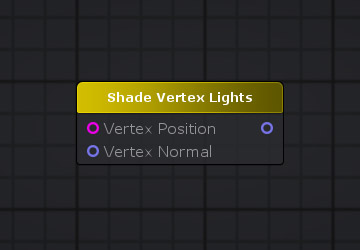The Shade Vertex Lights computes illumination from four per-vertex lights and ambient, given object space position and normal.

NOTE: This node only outputs correct results on template vertex/frag shaders with their LightMode set to Vertex.Node Parameter Description Default Value
Vertex Position Vertex position to be used on the light calculation. Only visible if the respective input port is not connected. 0,0,0,0
Vertex Normal Vertex normal to be used on the light calculation.. Only visible if the respective input port is not connected. 0,0,0
Light Amount Amount of nearest lights to take into account on the lighting calculation. This value can range from 0 to 8. 4
Is Spotlight Specify if lights are treated as spotlights ( more expensive ) or point lights. False
Input Port Description Type
Vertex Position Vertex position to be used on the light calculation. Float4
Vertex Normal Vertex normal to be used on the light calculation. Float3